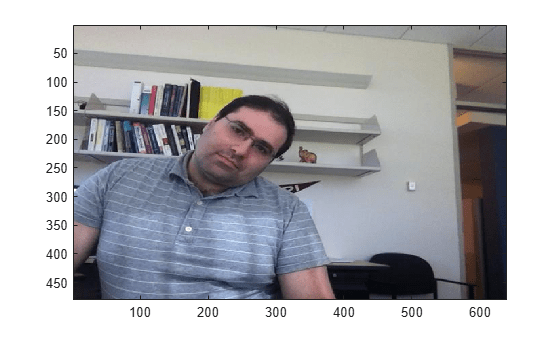# Perform Face Detection by Using OpenCV in MATLAB

This example shows how to detect faces in an image or video by using prebuilt MATLAB® interface to the OpenCV function `cv::CascadeClassifier`. This example uses a Harr face detection model that is trained for scale-invariant, frontal face detection. In this example, you also use the `createMat` utility function to define the input and output arrays, the `getImage` utility function to read the output image returned by the OpenCV function, and the `rectToBbox` utility function to convert the face detection output returned by the OpenCV function to bounding box coordinates in MATLAB®.

Read a video into the MATLAB workspace by using the `VideoReader` MATLAB function.

`videoSample = VideoReader("tilted_face.avi");`

Add the MATLAB interface to OpenCV package names to the import list.

```import clib.opencv.*; import vision.opencv.util.*;```

Specify the file name of a pre-trained trained Haar face detection model.

`trainedModel = "haarcascade_frontalface_alt.xml";`

Load the pre-trained model by using the `load` method of the OpenCV function `cv.CascadeClassifier`.

```cascadeClassify = cv.CascadeClassifier(); cascadeClassify.load(trainedModel);```

Specify the scale factor to use for multi-scale detection.

`scaleFactor = 1.2;`

Follow these steps to detect faces in each frame by using the `detectMultiScale` method of the OpenCV class `cv.CascadeClassifier`.

• Create a `Mat` object and store the input frame to the `Mat` object by using the `createMat` function. Specify the `Mat` object as an input to the `detectMultiScale` method.

• Create a MATLAB array to represent the OpenCV class for 2D rectangles `cv::Rect2i`. Specify the array as an input to the `detectMultiScale` method. The method uses the array to return the detection results.

• Export the detection results returned by the `detectMultiScale` method to a row vector by using the `rectToBbox` function. The row vector specifies bounding box coordinates in one-based indexing.

• Draw the bounding boxes on the input frame to represent the detected faces.

```count = 1; detections = cell(1,videoSample.NumFrames); while(hasFrame(videoSample)) testFrame = readFrame(videoSample); [inputMat,inputArray] = createMat(testFrame); results = clibArray("clib.opencv.cv.Rect2i", 0); cascadeClassify.detectMultiScale(inputArray,results,scaleFactor); if results.Dimensions ~= 0 detections{count} = rectToBbox(results); else detections{count} = []; end testFrame = insertShape(testFrame,Rectangle=detections{count},LineWidth=5); image(testFrame,Parent=gca); pause(0.01) count = count+1; end```